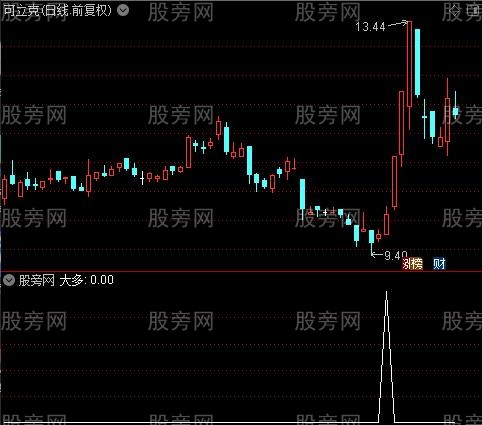# 长KDJ买卖提示之大多选股指标公式此处内容已被隐藏，请输入验证码查看内容。

SJ:=1;
N8:=80;
M18:=3;
M28:=3;
RSV8:=(CLOSE-LLV(LOW,N8))/(HHV(HIGH,N8)-LLV(LOW,N8))*100;
K98:=SMA(RSV8,M18,1);
D98:=SMA(K98,M28,1);
RK8:=MA(K98,80)*SJ;
RD8:=MA(D98,80)*SJ;### 相关文章